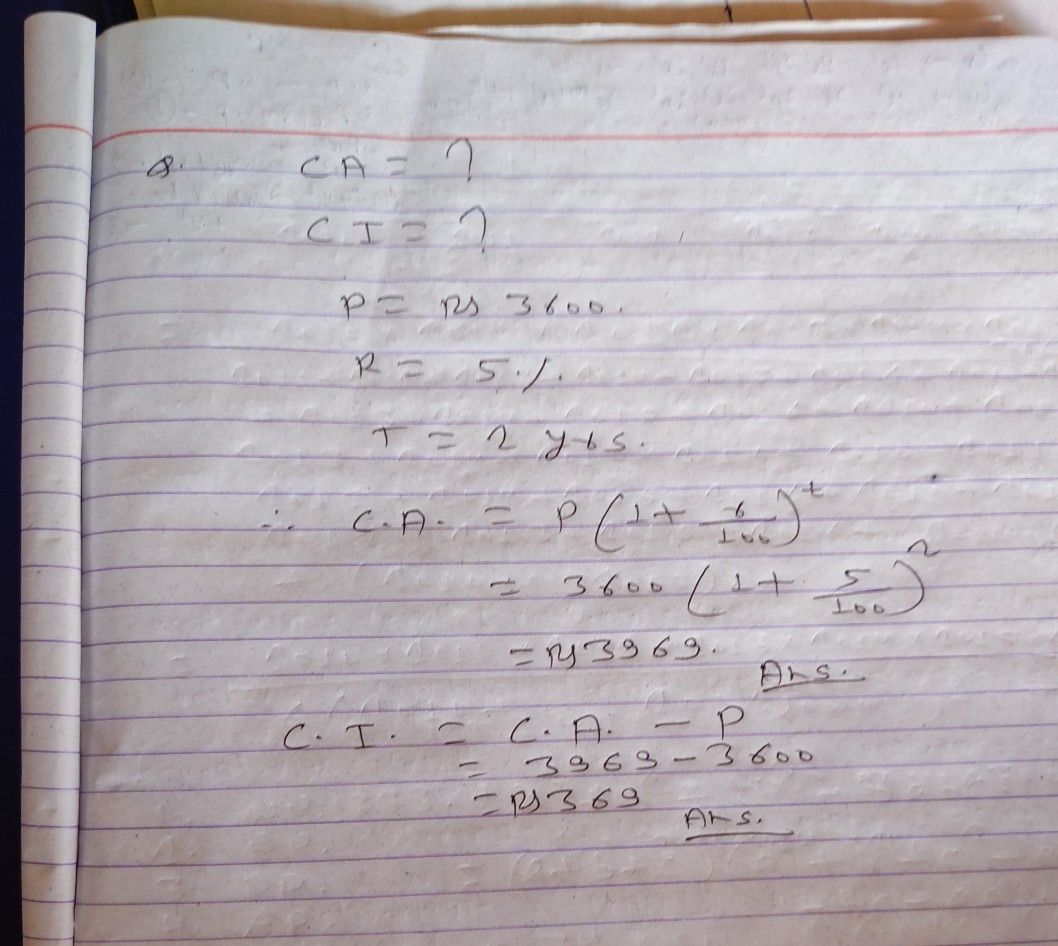Symbol
Problem$c0$ compounded annually for $2$ years. $Ca/cw$ Calculate the amount and compound interest by simple interest method $O\square$ $36OO$ $5%$ $p.a$ compounded annually for $2$ years. ulate the amount and the compound interest by simple interest method on $8$ $48OO$